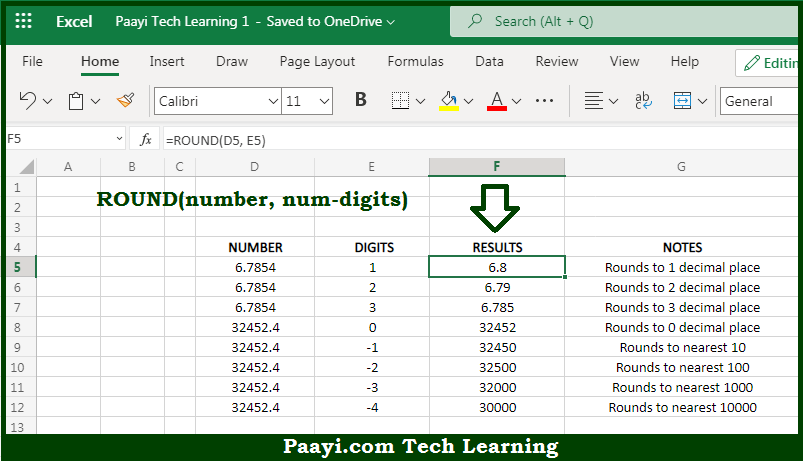# Learn How to Use Microsoft Excel ROUND Function

Written by | 0 Comments | 590 Views

In this article, you will learn how to use the Microsoft Excel ROUND function and its prime function in Microsoft Excel. You will also get to know the Microsoft Excel ROUND function return value and syntax with the help of some examples.

Microsoft Excel ROUND Function

The main function of the Microsoft Excel ROUND function is to round a number to the given number of digits. That implies, with the help of the ROUND function you can able to return a rounded number up to a given number of digits. It should be noted that The ROUND function can round to the right or left of the decimal point. So, with the help of the ROUND function, you can able to round a number to the given number of digits.

Return Value of ROUND Function

The return value will be the rounded number.

Syntax of ROUND Function

=ROUND(number, num-digits)

Where the arguments:

• number: This is the number which you want to round.
• num-digits: This is the number of digits the number should be rounded.

## How to Use Microsoft Excel ROUND Function?So we know that Microsoft Excel ROUND function you can able to round a number to the given number of digits. That implies, with the help of the ROUND function you can able to return a rounded number up to a given number of digits. It should be noted that The ROUND function can round to the right or left of the decimal point. So, with the help of the ROUND function, you can able to round a number to the given number of digits.